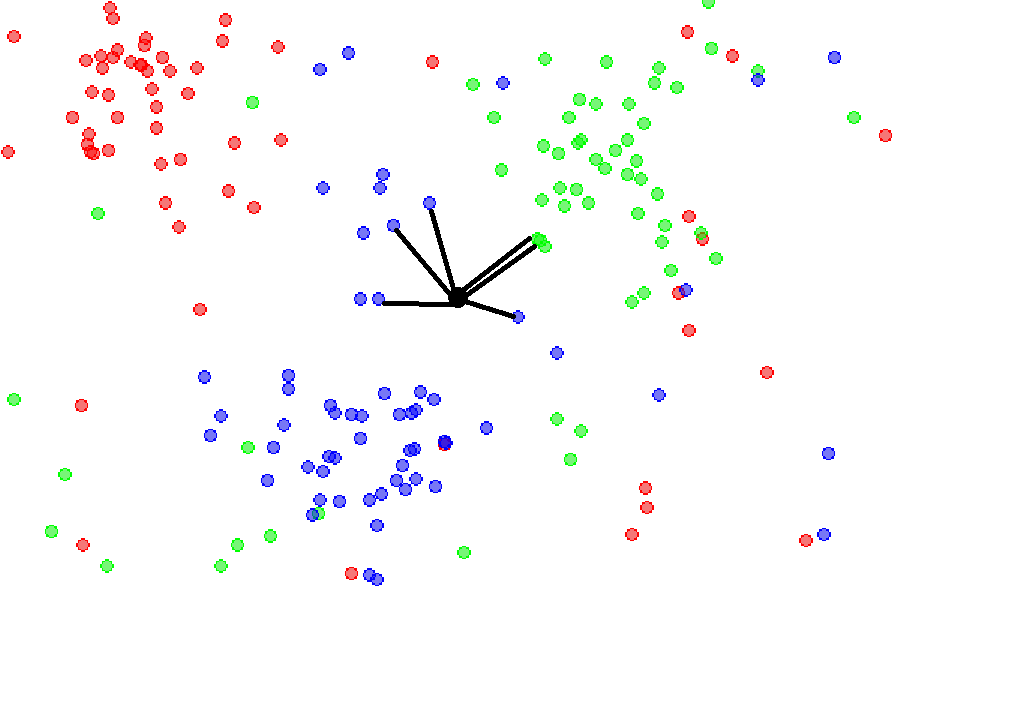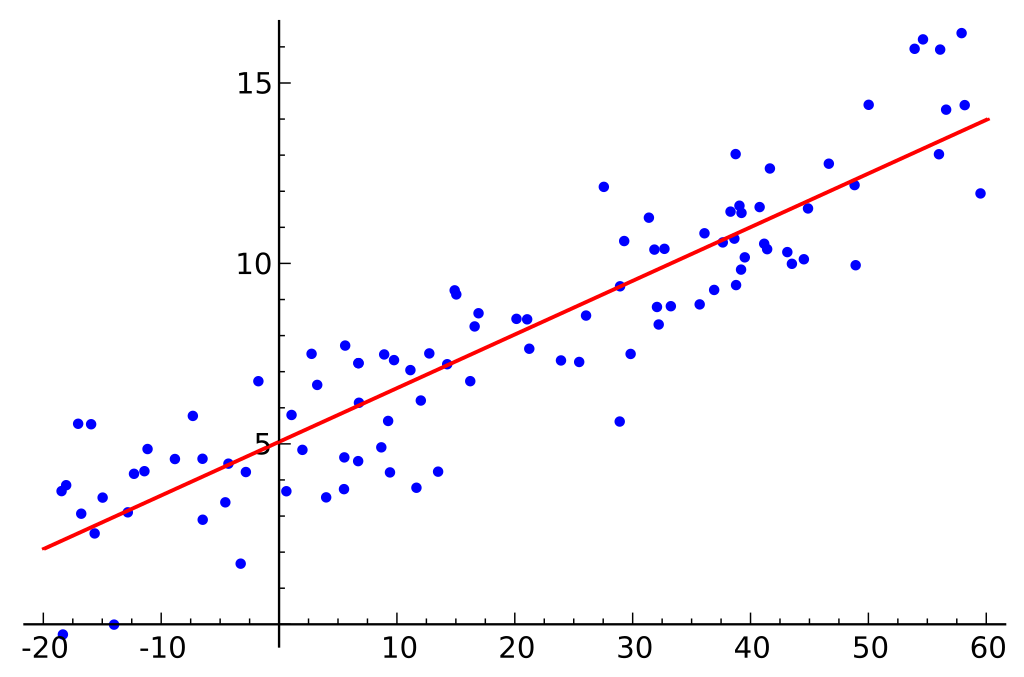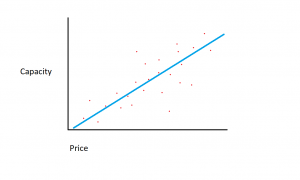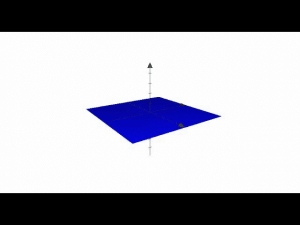Connect with us

# What is Reinforcement Learning?Published

onPut simply, reinforcement learning is a machine learning technique that involves training an artificial intelligence agent through the repetition of actions and associated rewards. A reinforcement learning agent experiments in an environment, taking actions and being rewarded when the correct actions are taken. Over time, the agent learns to take the actions that will maximize its reward. That’s a quick definition of reinforcement learning, but taking a closer look at the concepts behind reinforcement learning will help you gain a better, more intuitive understanding of it.

## Reinforcement In Psychology

The term “reinforcement learning” is adapted from the concept of reinforcement in psychology. For that reason, let’s take a moment to understand the psychological concept of reinforcement. In the psychological sense, the term reinforcement refers to something that increases the likelihood that a particular response/action will occur. This concept of reinforcement is a central idea of the theory of operant conditioning, initially proposed by the psychologist B.F. Skinner. In this context, reinforcement is anything that causes the frequency of a given behavior to increase. If we think about possible reinforcement for humans, these can be things like praise, a raise at work, candy, and fun activities.

In the traditional, psychological sense, there are two types of reinforcement. There’s positive reinforcement and negative reinforcement. Positive reinforcement is the addition of something to increase a behavior, like giving your dog a treat when it is well behaved. Negative reinforcement involves removing a stimulus to elicit a behavior, like shutting off loud noises to coax out a skittish cat.

## Positive and Negative Reinforcement In Machine Learning

Positive reinforcement increases the frequency of a behavior while negative reinforcement decreases the frequency. In general, positive reinforcement is the most common type of reinforcement used in reinforcement learning, as it helps models maximize the performance on a given task. Not only that but positive reinforcement leads the model to make more sustainable changes, changes which can become consistent patterns and persist for long periods of time.

In contrast, while negative reinforcement also makes a behavior more likely to occur, it is used for maintaining a minimum performance standard rather than reaching a model’s maximum performance. Negative reinforcement in reinforcement learning can help ensure that a model is kept away from undesirable actions, but it can’t really make a  model explore desired actions.

## Training A Reinforcement Agent

When a reinforcement learning agent is trained, there are four different ingredients or states used in the training: initial states (State 0), new state (State 1), actions, and rewards.

Imagine that we are training a reinforcement agent to play a platforming video game where the AI’s goal is to make it to the end of the level by moving right across the screen. The initial state of the game is drawn from the environment, meaning the first frame of the game is analyzed and given to the model. Based on this information, the model must decide on an action.

During the initial phases of training, these actions are random but as the model is reinforced, certain actions will become more common.  After the action is taken the environment of the game is updated and a new state or frame is created. If the action taken by the agent produced a desirable result, let’s say in this case that the agent is still alive and hasn’t been hit by an enemy, some reward is given to the agent and it becomes more likely to do the same in the future.

This basic system is constantly looped, happening again and again, and each time the agent tries to learn a little more and maximize its reward.

Reinforcement learning tasks can typically be placed in one of two different categories: episodic tasks and continual tasks.

Episodic tasks will carry out the learning/training loop and improve their performance until some end criteria are met and the training is terminated. In a game, this might be reaching the end of the level or falling into a hazard like spikes. In contrast, continual tasks have no termination criteria, essentially continuing to train forever until the engineer chooses to end the training.

## Monte Carlo vs Temporal Difference

There are two primary ways of learning, or training, a reinforcement learning agent. In the Monte Carlo approach, rewards are delivered to the agent (its score is updated) only at the end of the training episode. To put that another way, only when the termination condition is hit does the model learn how well it performed. It can then use this information to update and when the next training round is started it will respond in accordance to the new information.

The temporal-difference method differs from the Monte Carlo method in that the value estimation, or the score estimation, is updated during the course of the training episode. Once the model advances to the next time step the values are updated.

## Explore vs Exploit

Training a reinforcement learning agent is a balancing act, involving the balancing of two different metrics: exploration and exploitation.

Exploration is the act of collecting more information about the surrounding environment, while exploration is using the information already known about the environment to earn reward points. If an agent only explores and never exploits the environment, the desired actions will never be carried out. On the other hand, if the agent only exploits and never explores, the agent will only learn to carry out one action and won’t discover other possible strategies of earning rewards. Therefore, balancing exploration and exploitation is critical when creating a reinforcement learning agent.

## Uses For Reinforcement Learning

Reinforcement learning can be used in a wide variety of roles, and it is best suited for applications where tasks require automation.

Automation of tasks to be carried out by industrial robots is one area where reinforcement learning proves useful. Reinforcement learning can also be used for problems like text mining, creating models that are able to summarize long bodies of text. Researchers are also experimenting with using reinforcement learning in the healthcare field, with reinforcement agents handling jobs like the optimization of treatment policies. Reinforcement learning could also be used to customize educational material for students.

## Concluding Thoughts

Reinforcement learning is a powerful method of constructing AI agents that can lead to impressive and sometimes surprising results. Training an agent through reinforcement learning can be complex and difficult, as it takes many training iterations and a delicate balance of the explore/exploit dichotomy. However, if successful, an agent created with reinforcement learning can carry out complex tasks under a wide variety of different environments.

Recommended Reinforcement Learning CoursesOffered ByDurationDifficulty

Introduction to Artificial Intelligence

IBM

9 Hours

Beginner

Fundamentals of Reinforcement Learning

University of Alberta

18 Hours

Beginner

Machine Learning Foundations

University of Washington

24 Hours

Intermediate

Reinforcement Learning Specialization

University of Alberta

3 Months

IntermediateBlogger and programmer with specialties in Machine Learning and Deep Learning topics. Daniel hopes to help others use the power of AI for social good.

# AI Algorithms Used To Develop Drugs That Fight Drug-Resistant BacteriaPublished

onOne of the biggest challenges facing the medical industry is drug-resistant bacteria. Currently, there are some estimated 700,000 deaths due to drug-resistant bacteria, and more strains of drug-resistant bacteria are developing. Scientists and engineers are attempting to develop new methods of combatting drug-resistant bacteria. One method of developing new antibiotics is employing artificial intelligence and machine learning to isolate new compounds that could deal with new strains of super-bacteria.

As SingularityHub reported, a new antibiotic was designed with the assistance of AI. The antibiotic has been named halicin, after the AI HAL from 2001: A Space Odyssey. The newly developed antibiotic proved successful at eliminating some of the virile super-bacteria strains. The new antibiotic was discovered through the use of machine learning algorithms. Specifically, the machine learning model was trained using a large dataset comprised of approximately 2,500 compounds. Nearly half of the drugs used to train the model were drugs already approved by the FDA, while the other half of the training set was comprised of naturally occurring compounds. The team of researchers tweaked the algorithms to prioritize molecules that simultaneously possessed antibiotic properties but different from existing antibiotic structures. They then examined the results to determine which compounds would be safe for human consumption.

According to The Guardian, the drug proved extremely effective at fighting drug-resistant bacteria in a recent study. It is so effective because it degrades the membrane of the bacteria, which disables the ability of the bacteria to produce energy. For bacteria to develop defenses against the effects of halicin it could take more than a few genetic mutations, which gives halicin staying power. The research team also tested how the compound performed in mice, where it was able to successfully clear mice infected with a strain of bacteria resistant to all current antibiotics. With the results of the studies so promising, the research team is hoping to move into a partnership with a pharmaceutical entity and prove the drug safe for use by people.

James Collins, professor of bioengineering and senior author at MIT, and Regina Barzilay, computer science professor at MIT were both senior authors on the paper. Collins, Barzilay, and other researchers hope that algorithms like the type they used to design halicin could help fast-track the discovery of new antibiotics to deal with the proliferation of drug-resistant strains of the disease.

Halicin is far from the only drug compound discovered with the use of AI. The research team lead by Collin and Barzilay want to go farther and create new compounds training more models using around 100 million molecules pulled from the ZINC 15 database, an online library of over 1.5 billion drug compounds. Reportedly the team has already managed to find at least 23 different candidates that satisfy the criteria of being possibly safe for human use and structurally different from current antibiotics.

An unfortunate side effect of antibiotics is that, while they kill harmful bacteria, they also kill off the necessary gut bacteria that the human body needs. The research hopes that they could use techniques similar to the those used to create halicin to create antibiotics with fewer side effects, drugs less likely to harm the human gut microbiome.

Many other companies are also attempting to use machine learning to simplify the complex, long, and often expensive drug creation process. Other companies have also been training AI algorithms to synthesize new drug compounds. Just recently one company was able to develop a proof-of-concept drug in only a month and a half, a much shorter amount of time than the months or even years it can take to create a drug the traditional way.

Barzilay is optimistic that AI-driven drug discovery methods can transform the landscape of drug discovery in meaningful ways. Barzilay explained that the work on halicin is a practical example of how effective machine learning techniques can be:

“There is still a question of whether machine-learning tools are really doing something intelligent in healthcare, and how we can develop them to be workhorses in the pharmaceuticals industry. This shows how far you can adapt this tool.”

# What is K-Nearest Neighbors?Published

onK-Nearest Neighbors is a machine learning technique and algorithm that can be used for both regression and classification tasks. K-Nearest Neighbors examines the labels of a chosen number of data points surrounding a target data point, in order to make a prediction about the class that the data point falls into. K-Nearest Neighbors (KNN) is a conceptually simple yet very powerful algorithm, and for those reasons, it’s one of the most popular machine learning algorithms. Let’s take a deep dive into the KNN algorithm and see exactly how it works. Having a good understanding of how KNN operates will let you appreciated the best and worst use cases for KNN.

## An Overview Of KNNPhoto: Antti Ajanki AnAj via Wikimedia Commons, CC BY SA 3.0 (https://commons.wikimedia.org/wiki/File:KnnClassification.svg)

Let’s visualize a dataset on a 2D plane. Picture a bunch of data points on a graph, spread out along the graph in small clusters. KNN examines the distribution of the data points and, depending on the arguments given to the model, it separates the data points into groups. These groups are then assigned a label. The primary assumption that a KNN model makes is that data points/instances which exist in close proximity to each other are highly similar, while if a data point is far away from another group it’s dissimilar to those data points.

A KNN model calculates similarity using the distance between two points on a graph. The greater the distance between the points, the less similar they are. There are multiple ways of calculating the distance between points, but the most common distance metric is just Euclidean distance (the distance between two points in a straight line).

KNN is a supervised learning algorithm, meaning that the examples in the dataset must have labels assigned to them/their classes must be known. There are two other important things to know about KNN. First, KNN is a non-parametric algorithm. This means that no assumptions about the dataset are made when the model is used. Rather, the model is constructed entirely from the provided data. Second, there is no splitting of the dataset into training and test sets when using KNN. KNN makes no generalizations between a training and testing set, so all the training data is also used when the model is asked to make predictions.

## How The KNN Algorithm Operates

A KNN algorithm goes through three main phases as it is carried out:

1. Setting K to the chosen number of neighbors.
2. Calculating the distance between a provided/test example and the dataset examples.
3. Sorting the calculated distances.
4. Getting the labels of the top K entries.
5. Returning a prediction about the test example.

In the first step, K is chosen by the user and it tells the algorithm how many neighbors (how many surrounding data points) should be considered when rendering a judgment about the group the target example belongs to. In the second step, note that the model checks the distance between the target example and every example in the dataset. The distances are then added into a list and sorted. Afterward, the sorted list is checked and the labels for the top K elements are returned. In other words, if K is set to 5, the model checks the labels of the top 5 closest data points to the target data point. When rendering a prediction about the target data point, it matters if the task is a regression or classification task. For a regression task, the mean of the top K labels is used, while the mode of the top K labels is used in the case of classification.

The exact mathematical operations used to carry out KNN differ depending on the chosen distance metric. If you would like to learn more about how the metrics are calculated, you can read about some of the most common distance metrics, such as Euclidean, Manhattan, and Minkowski.

## Why The Value Of K Matters

The main limitation when using KNN is that in an improper value of K (the wrong number of neighbors to be considered) might be chosen. If this happen, the predictions that are returned can be off substantially. It’s very important that, when using a KNN algorithm, the proper value for K is chosen. You want to choose a value for K that maximizes the model’s ability to make predictions on unseen data while reducing the number of errors it makes.Photo: Agor153 via Wikimedia Commons, CC BY SA 3.0 (https://en.wikipedia.org/wiki/File:Map1NN.png)

Lower values of K mean that the predictions rendered by the KNN are less stable and reliable. To get an intuition of why this is so, consider a case where we have 7 neighbors around a target data point. Let’s assume that the KNN model is working with a K value of 2 (we’re asking it to look at the two closest neighbors to make a prediction). If the vast majority of the neighbors (five out of seven) belong to the Blue class, but the two closest neighbors just happen to be Red, the model will predict that the query example is Red. Despite the model’s guess, in such a scenario Blue would be a better guess.

If this is the case, why not just choose the highest K value we can? This is because telling the model to consider too many neighbors will also reduce accuracy. As the radius that the KNN model considers increases, it will eventually start considering data points that are closer to other groups than they are the target data point and misclassification will start occurring. For example, even if the point that was initially chosen was in one of the red regions above, if K was set too high, the model would reach into the other regions to consider points. When using a KNN model, different values of K are tried to see which value gives the model the best performance.

## KNN Pros And Cons

Let’s examine some of the pros and cons of the KNN model.

Pros:

KNN can be used for both regression and classification tasks, unlike some other supervised learning algorithms.

KNN is highly accurate and simple to use. It’s easy to interpret, understand, and implement.

KNN doesn’t make any assumptions about the data, meaning it can be used for a wide variety of problems.

Cons:

KNN stores most or all of the data, which means that the model requires a lot of memory and its computationally expensive. Large datasets can also cause predictions to be take a long time.

KNN proves to be very sensitive to the scale of the dataset and it can be thrown off by irrelevant features fairly easily in comparison to other models.

## Summing Up

K-Nearest Neighbors is one of the simplest machine learning algorithms. Despite how simple KNN is, in concept, it’s also a powerful algorithm that gives fairly high accuracy on most problems. When you use KNN, be sure to experiment with various values of K in order to find the number that provides the highest accuracy.

# What is Linear Regression?Published

onLinear regression is an algorithm used to predict, or visualize, a relationship between two different features/variables. In linear regression tasks, there are two kinds of variables being examined: the dependent variable and the independent variable. The independent variable is the variable that stands by itself, not impacted by the other variable. As the independent variable is adjusted, the levels of the dependent variable will fluctuate. The dependent variable is the variable that is being studied, and it is what the regression model solves for/attempts to predict. In linear regression tasks, every observation/instance is comprised of both the dependent variable value and the independent variable value.

That was a quick explanation of linear regression, but let’s make sure we come to a better understanding of linear regression by looking at an example of it and examining the formula that it uses.

## Understanding Linear Regression

Assume that we have a dataset covering hard-drive sizes and the cost of those hard drives.

Let’s suppose that the dataset we have is comprised of two different features: the amount of memory and cost. The more memory we purchase for a computer, the more the cost of the purchase goes up. If we plotted out the individual data points on a scatter plot, we might get a graph that looks something like this:The exact memory-to-cost ratio might vary between manufacturers and models of hard drive, but in general, the trend of the data is one that starts in the bottom left (where hard drives are both cheaper and have smaller capacity) and moves to the upper right (where the drives are more expensive and have higher capacity).

If we had the amount of memory on the X-axis and the cost on the Y-axis, a line capturing the relationship between the X and Y variables would start in the lower-left corner and run to the upper right.The function of a regression model is to determine a linear function between the X and Y variables that best describes the relationship between the two variables. In linear regression, it’s assumed that Y can be calculated from some combination of the input variables. The relationship between the input variables (X) and the target variables (Y) can be portrayed by drawing a line through the points in the graph. The line represents the function that best describes the relationship between X and Y (for example, for every time X increases by 3, Y increases by 2). The goal is to find an optimal “regression line”, or the line/function that best fits the data.

Lines are typically represented by the equation: Y = m*X + b. X refers to the dependent variable while Y is the independent variable. Meanwhile, m is the slope of the line, as defined by the “rise” over the “run”. Machine learning practitioners represent the famous slope-line equation a  little differently, using this equation instead:

y(x) = w0 + w1 * x

In the above equation, y is the target variable while “w” is the model’s parameters and the input is “x”. So the equation is read as: “The function that gives Y, depending on X, is equal to the parameters of the model multiplied by the features”. The parameters of the model are adjusted during training to get the best-fit regression line.

## Multiple RegressionPhoto: Cbaf via Wikimedia Commons, Public Domain (https://commons.wikimedia.org/wiki/File:2d_multiple_linear_regression.gif)

The process described above applies to simple linear regression, or regression on datasets where there is only a single feature/independent variable. However, a regression can also be done with multiple features. In the case of “multiple linear regression”, the equation is extended by the number of variables found within the dataset. In other words, while the equation for regular linear regression is y(x) = w0 + w1 * x, the equation for multiple linear regression would be y(x) = w0 + w1x1 plus the weights and inputs for the various features. If we represent the total number of weights and features as w(n)x(n), then we could represent the formula like this:

y(x) = w0 + w1x1 + w2x2 + … + w(n)x(n)

After establishing the formula for linear regression, the machine learning model will use different values for the weights, drawing different lines of fit. Remember that the goal is to find the line that best fits the data in order to determine which of the possible weight combinations (and therefore which possible line) best fits the data and explains the relationship between the variables.

A cost function is used to measure how close the assumed Y values are to the actual Y values when given a particular weight value. The cost function for linear regression is mean squared error, which just takes the average (squared) error between the predicted value and the true value for all of the various data points in the dataset. The cost function is used to calculate a cost, which captures the difference between the predicted target value and the true target value. If the fit line is far from the data points, the cost will be higher, while the cost will become smaller the closer the line gets to capturing the true relationships between variables. The weights of the model are then adjusted until the weight configuration that produces the smallest amount of error is found.